# Maxwell's Equations

Also found in: Dictionary, Thesaurus, Wikipedia.
Related to Maxwell's Equations: James Clerk Maxwell

## Maxwell's equations

Four differential equations proposed by James Clerk Maxwell in 1864 as the basis of the theory of electromagnetic waves. They may be written, in vector notation, as Eqs. (1)–(4), where D is the electric

(1)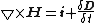(2)(3)(4)displacement, B the magnetic flux density, E the electric field strength or intensity, H the magnetic field strength or intensity, ρ the charge density, and i the current density.

The first equation states that electric flux lines, if they end at all, will do so on electric charges. The second states that magnetic flux lines never terminate. The third is a form of Faraday's law of induction, which states that the rate of change of the magnetic flux threading a circuit equals the electromotive force or line integral of E around the circuit. The fourth integral is based partially on A. M. Ampère's experiments on steady currents which show that the line integral of the magnetic intensity H (or B /μ, where μ is the permeability) around a closed curve equals the current encircled. See Displacement current

McGraw-Hill Concise Encyclopedia of Physics. © 2002 by The McGraw-Hill Companies, Inc.
The following article is from The Great Soviet Encyclopedia (1979). It might be outdated or ideologically biased.

## Maxwell’s Equations

the fundamental equations of classical macroscopic electrodynamics that describe electromagnetic phenomena in any medium. The equations were formulated by J. C. Maxwell in the 1860’s on the basis of a generalization of the empirical laws of electric and magnetic phenomena. By using these laws as a basis and developing M. Faraday’s productive idea that the interactions between electrically charged bodies take place through an electromagnetic field, Maxwell created the theory of electromagnetic processes, which is expressed mathematically by Maxwell’s equations. The present form of the equations was given by the German physicist H. Hertz and the British physicist O. Heaviside.

Maxwell’s equations relate the quantities that characterize an electromagnetic field to its sources, that is, to the spatial distribution of electric charges and currents. In a vacuum, the electromagnetic field is characterized by two vector quantities that are dependent on spatial coordinates and on time—the electric field intensity E and magnetic induction B. These quantities determine the forces that act because of the field on the charges and currents whose distribution in space is given by the charge density ρ (the charge per unit volume) and the current density j (the charge passing in unit time through unit area perpendicular to the direction of motion of the charges). In addition to the vectors E and B, auxiliary vector quantities that are dependent on the state and properties of the medium—the electric displacement D and the magnetic field intensity H—are introduced to describe electromagnetic processes in a material medium (matter).

Maxwell’s equations make it possible to determine the fundamental characteristics of a field (E, B, D, and H) at each point in space at any moment if the field sources j and ρ are known as functions of the coordinates and of time. The equations can be written in integral or differential form [below they are given in the absolute (Gaussian) system of units].

Maxwell’s equations in integral form determine on the basis of given charges and currents not the field vectors E, B, D and H themselves at different points in space but certain integral quantities that depend on the distribution of these field characteristics: the line integral (circulation) of the vectors E and H around any closed curve and the surface integral (flux) of the vectors D and B through any closed surface.

Maxwell’s first equation is a generalization for variable fields of the empirical law of Ampere which deals with the excitation of a magnetic field by an electric current. Maxwell advanced the hypothesis that magnetic fields are generated not only by currents flowing in conductors but also by varying electric fields in dielectrics or a vacuum. A quantity proportional to the rate of change of the electric field with time was called displacement current by Maxwell. A displacement current excites a magnetic field by the same law as does a conduction current (this was confirmed later experimentally). The total current, which is equal to the sum of the conduction current and the displacement current, is always closed.

Maxwell’s first equation has the form:that is, the line integral of the magnetic field vector around a closed curve L (the sum of the scalar products of the vector H at a given point in the circuit and an infinitesimal segment dl of the curve) is given by the total current through any surface S bounded by the curve. Here jn is the projection of the conduction current density j on the normal to the infinitesimal area ds, which is a part of the surface S; (1/4π)(∂Dn/∂t) is the projection of the displacement current density on the same normal; and c = 3 × 1010 cm/sec is a constant equal to the rate of propagation of electromagnetic interactions in a vacuum.

Maxwell’s second equation is a mathematical formulation of Faraday’s law of elecromagnetic induction and is written in the form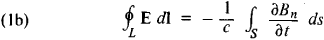that is, the line integral of the electric field vector along a closed curve L (the electromotive force of induction) is given by the rate of change of the magnetic induction through the surface S bounded by the curve. Here Bn is the projection of the magnetic induction vector B on the normal to the area ds; the minus sign corresponds to the Lenz law for the direction of an induced current.

Maxwell’s third equation expresses the experimental findings on the absence of magnetic charges analogous to electric charges (a magnetic field is produced only by currents):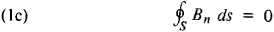that is, the magnetic induction through any closed surface S is equal to zero.

Maxwell’s fourth equation, which is usually called Gauss’ theorem, is a generalization of the law of interaction of stationary electric charges — Coulomb’s law: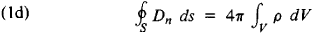that is, the electric flux through an arbitrary closed surface S is determined by the electric charge located within this surface (in the volume V bounded by the given surface).

If we assume that the vectors of an electromagnetic field (E, B, D, H) are continuous functions of the coordinates, then, by considering the line integrals of the vectors H and E around infinitesimal closed curves and the surface integrals of the vectors B and D through surfaces that bound infinitesimal volumes, we can proceed from the integral relations (1a)-(1d) to a system of differential equations that are valid at any point in space, that is, we can obtain a differential form of Maxwell’s equations (which is usually more convenient for solving problems):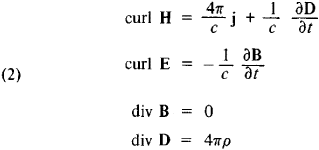Here curl and div are the differential operators known as curl and divergence, which act on the vectors H, E, B, and D. The physical import of equations (2) is the same as that of equations (1a)-(1d).

Maxwell’s equations in form (1a)-(1d) or (2) do not form a complete closed system that would allow calculation of electromagnetic processes in the presence of a material medium. They must be supplemented by relations between the vectors E, H, D, B, and j, which are not independent. The relations among these vectors are determined by the properties and state of the medium; D and j are expressed in terms of E, and B is expressed in terms of H:

(3) D = D(E), B = B(H), j = j(E)

These three equations are called the constitutive relations, or the material equations; they describe the electromagnetic properties of the medium and have a definite form for a specific medium. In a vacuum, DE and BH. The set of field equations (2) and constitutive relations (3) forms the complete system of equations.

Maxwell’s macroscopic equations describe a medium phenomenologically, without examining the complex mechanism of the interaction of the electromagnetic field with the charged particles of the medium. Maxwell’s equations can be obtained from the Lorentz-Maxwell equations for microscopic fields and for specific conceptions of the structure of matter by averaging the microfields over small space-time intervals. The basic field equations (2) and the specific form of the constitutive relations (3) are obtained in this manner, and the form of the field equations is independent of the properties of the medium.

The constitutive relations are very complex in the general case, since the vectors D, B, and j at a given point in space and a given moment may depend on the fields E and H at all points in the medium and at all previous moments of time. In some media the vectors D and B may be nonzero with E and H equal to zero (ferroelectrics and ferromagnetics). However, for the majority of isotropic media and up to extremely large fields, the equations of state have the simple linear form

(4) D = εE, B = μH, j = σE + jnon

Here, ε(x,y,z) is the dielectric constant and μ(x,y,z) is the permeability of the medium, which characterize its electric and magnetic properties, respectively (in the selected system of units ε = μ = 1 for a vacuum); the quantity σ(x,y,z) is called the specific electric conductivity; and jnon is the density of nonelectromagnetic currents, that is, currents maintained by any forces other than those of an electric field (such as a magnetic field or diffusion). In Maxwell’s phenomenological theory, the macroscopic characteristics ε, μ, and σ of the electromagnetic properties of the medium must be found experimentally. In the Lorentz-Maxwell microscopic theory they can be calculated.

The dielectric constant ε and permeability μ actually determine the contribution to the electromagnetic field by bound charges, which are part of the electrically neutral atoms and molecules of matter. The experimental determination of ε, μ, and σ makes it possible to calculate the electromagnetic field in a medium without solving the difficult auxiliary problem of the distribution of bound charges and of the corresponding currents in the substance. In Maxwell’s equations the charge density ρ and the current density j are the densities of free charges and currents, and the auxiliary vectors H and D are introduced such that the circulation of the vector H is determined only by the motion of free charges, and the flux of vector D by the density of the distribution of these charges in space.

If the electromagnetic field is considered in two adjacent media, then the field vectors at their interface may experience discontinuities. In this case, equations (2) must be supplemented by the boundary conditions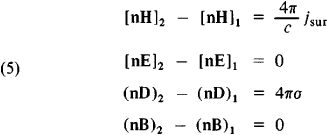Here jsur and σ are the densities of the surface current and charge, the brackets and parentheses represent, respectively, the vector and scalar products of the vectors, n is the unit vector along the normal to the interface in the direction of the second medium from the first (1 → 2), and the subscripts refer to the different sides of the interface.

The basic field equations (2) are linear, while the material equations (3) may also be nonlinear. Nonlinear effects are usually observed in quite strong fields. In linear media [satisfying relations (4)] and, in particular, in a vacuum, Maxwell’s equations are linear and thus the principle of superposition is valid: when the fields are superimposed they do not affect each other.

A number of laws of conservation stem from Maxwell’s equations. In particular, we can derive from equations (1a) and (1d) the relation (called the equation of continuity)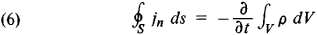which is the law of conservation of electric charge: the total current flowing through any closed surface S per unit time is equal to the rate of change of the charge within the volume V bounded by this surface. If there is no current through the surface, then the charge in the volume remains unchanged.

It follows from Maxwell’s equations that an electromagnetic field has energy and momentum. The energy density w (the energy per unit volume of the field) is equal toElectromagnetic energy can travel in space. The energy flux density is given by the Poynting vector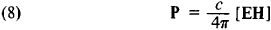The direction of the Poynting vector is perpendicular to both E and H and coincides with the direction of propagation of the electromagnetic energy, and its magnitude is equal to the energy passing per unit time through unit area of surface perpendicular to the vector P. If no conversions of electromagnetic energy into other forms take place, then, according to Maxwell’s equations, the change in energy in any volume per unit time is equal to the flux of electromagnetic energy through the surface that bounds this volume. If heat is released within the volume at the expense of electromagnetic energy, then the law of conservation of energy is written in the formwhere Q is the quantity of heat released per unit time.

The density of electromagnetic field momentum g (the momentum per unit volume of a field) is related to the energy flux density by the expressionThe existence of electromagnetic field momentum was first detected by P. N. Lebedev in experiments on the measurement of the pressure of light (1899).

As can be seen from (7), (8), and (10), an electromagnetic field always has energy, but the energy flux and electromagnetic momentum are nonzero only when both electric and magnetic fields exist simultaneously (and these fields are not parallel to each other).

Maxwell’s equations lead to the fundamental conclusion of the finiteness of the speed of propagation of electromagnetic interactions (equal to c = 3 × 1010 cm/sec). This means that when the charge or flux density changes at some point in space, the electromagnetic field that it generates does not change at the point of observation at the same moment but rather after a time τ = R/c, where R is the distance from the element of current or charge to the point of observation. The existence of electromagnetic waves, of which light waves are a particular case (as Maxwell first demonstrated), is possible because of the finite rate of propagation of electromagnetic interactions.

Electromagnetic phenomena take place in the same way in all inertial frames of reference, that is, they satisfy the principle of relativity. Accordingly, Maxwell’s equations do not change their form in going over from one inertial frame of reference to another (they are relativistically invariant). Satisfaction of the principle of relativity for electromagnetic processes proved to be incompatible with classical concepts of space and time. The required revision of these concepts led to the creation of the special theory of relativity (A. Einstein, 1905). The form of Maxwell’s equations remains unchanged in going over to a new inertial frame of reference if the spatial coordinates, time, the field vectors E, H, B, and D, current density j, and charge density ρ change in accordance with the Lorentz transformation (which expresses new, relativistic concepts of space and time). The relativistically invariant form of Maxwell’s equations underscores the fact that electric and magnetic fields form a unified whole.

Maxwell’s equations describe an enormous range of phenomena. They underlie electric and radio technology and play a very important role in the development of such significant areas of modern physics as plasma physics, the problem of controlled thermonuclear reactions, magnetohydrodynamics, nonlinear optics, the design of charged-particle accelerators, and astrophysics. Maxwell’s equations are inapplicable only for electromagnetic waves of high frequencies, when quantum effects become significant, that is, when the energy of the individual quanta of the electromagnetic field—photons—is large and a comparatively small number of photons take part in the processes.

### REFERENCES

Maxwell, J. C. Izbr. soch. po teorii elektromagnitnogo polia. Moscow, 1952. (Translated from English.)
Tamm, I. E. Osnovy teorii elektrichestva, 7th ed. Moscow, 1957.
Kalashnikov, S. G. Elektrichestvo. Moscow, 1956. (Obshchii kurs fiziki, vol. 2.)
Feynman, R., R. Leighton, and M. Sands. Feinmanovskie lektsiipofizike, fascs. 5, 6, 7. Moscow, 1966. (Translated from English.)
Landau, L. D., and E. M. Lifshits. Teoriia polia, 5th ed. Moscow, 1967. (Teoreticheskaia fizika, vol. 2.)
Landau, L. D., and E. M. Lifshits. Elektrodinamika sploshnykh sred. Moscow, 1959.

G. IA. MIAKISHEV

References in periodicals archive ?
The integral form of Maxwell's equations is given by:
Maxwell's equations encompass a wide range of applications and can describe electrical properties (2) of interconnects.
The primary field is assumed to be a monochromatic plane wave with irradiance [E.sub.0], wave-length [lambda], and circular wave number k = 2[pi]/[lambda] that is normally incident from the half space z<0 and is plane polarized so that, in accordance with Maxwell's equations, [partial]U/[partial]z or U are continuous and equal to zero on crossing the screen.
Yung, "The unconditionally stable crank-nicolson FDTD method for three-dimensional maxwell's equations," Microwave and Optical Technology Letters, vol.
The results presented here can be used to construct postprocessing procedures for the [H.sup.1]-nodal finite-element treatment of Maxwell's equations in domains with geometric singularities and with optimal accuracy.
Liu, "A 3-D discontinuous spectral element time-domain method for Maxwell's equations," IEEE Trans.
The effective coefficients in the quasi-steady Maxwell's equations for a multiscale isotropic medium are described in .
Conventional theory based on Maxwell's equations in the vacuum is symmetric in respect to the field strengths E and B.
Classically, it discretizes both the electric and magnetic fields in a staggered fashion on a finite cubic grid and approximates the derivatives occurring in Maxwell's equations by second-order accurate central differences.
Among their topics are magnetic and electromagnetic phenomena, modeling mechanical fluid systems, Maxwell's equations, tracking control of an electromechanical systems, and control of a multibody system's response to a suddenly applied force.
This theory is summarized by Maxwell's equations [3, 4]--the partial differential equations of EM.

Site: Follow: Share:
Open / Close